## Programming Models for Deep Learning and Deep Learning Frameworks¶

incl. explaination of Backpropagation and Autodiff

with examples in Theano and TensorFlow

#### Model specification¶

(from mxnet documentation)

• per configuration
• inflexible, just reuse of common building blocks
• examples: Caffe, CNTK
• programmatic
• flexible, programming of building blocks possible
• examples: Torch, Theano, Tensorflow, MXnet

### Two (programmatic) approaches:¶

(from mxnet documentation)

• imperative
• symbolic

### Imperative¶

Imperative style programs conduct the computation as we run them.

In :
import numpy as np
a = np.ones(10)
b = np.ones(10) * 2
c = b * a # when the programs execute to c = b * a,
#it runs the actual computation.
d = c + 1


#### Symbolic¶

A = Variable('A')
B = Variable('B')
C = B * A
D = C + Constant(1)
# compiles the function
f = compile(D)
d = f(A=np.ones(10), B=np.ones(10)*2)

#### Computational Graph¶

The difference in symbolic programs is when C = B * A is executed, there is no actual computation happening. Instead, these operations generate a computation graph (symbolic graph) that represents the computation it described.

• A and B are symbolic variables or symbols
In :
from IPython.display import Image
Image(filename='./pics/comp_graph.png', height=400)
# image from the mxnet documentation

Out: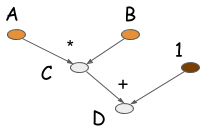Most symbolic style programs contain, either explicitly or implicitly, a compile step.

#### Theano¶

In :
import theano.tensor as T
from theano import function

# in theano each symbolic variable must be assigned a tensor (shape) type and data type
A = T.dscalar('A') # default of dtype is floatX
print ("A.dtype:", A.dtype)
B = T.dscalar('B')
C = T.mul(B, A)
D = C + 1
f = function(inputs=[A, B], outputs=D) # compile a function

A.dtype: float64

In :
f(1,2)

Out:
array(3.0)
In :
# implicit compilation with theano
Z.eval({A:2., B:5.})

Out:
array(21.0)

#### Tensorflow¶

In :
import tensorflow as tf

# if no graph is specified the default graph is used
# tf.placeholder for defining symbolic variables
A = tf.placeholder(name="A", dtype=tf.float32)
B = tf.placeholder(name="B", dtype=tf.float32)
C = tf.multiply(B, A, name="multiplication")
D = C + 1

In :
with tf.Session() as sess:
result = sess.run([D], feed_dict={A:1., B:[2.]})
print(result)

[array([ 3.], dtype=float32)]

In :
tf.get_variable?


#### Graph in Tensorflow¶

In :
g = tf.get_default_graph()
[op.name for op in g.get_operations()]

Out:
['A', 'B', 'multiplication', 'add/y', 'add']
In :
# write the definition of the graph to the log file
writer = tf.summary.FileWriter("./tmp/graph_logs",
tf.get_default_graph())
writer.flush()


Lanch tensorboard on the commandline:

>tensorboard --logdir=tmp/graph_logs/

Starting TensorBoard 23 on port 6006
(You can navigate to http://0.0.0.0:6006)#### Shared Variables¶

In Neural Networks learning is (mostly) optimization of parameters.

Values of variables are hold during execution of the computational graphs.

e.g. with a numpy array W_value.

• Theano: Shared Variables
weights = theano.shared(value=W_values, name='weights')

• Tensorflow: Variables
weights = tf.Variable(initial_value=W_values, name='weights') create a new variable every time it is called
or
weights = tf.get_variable(name='weights', initializer= ) also be used for weight sharing

#### Name and Variable Scope¶

Note: In tensorflow the names of variables and operators can be automatically prefixed (hierarchically) by

with tf.name_scope("first_layer") as scope:
...



The names of the variables (tf.get_variable(..)!) (and operators) can be automatically prefixed (hierarchically) by

with tf.variable_scope("first_layer") as scope:
...


• reuse=False: gives an exception if a variable name is used again.
• reuse=True: for sharing weights.

for the difference between tf.name_scope and tf.variable_scope see e.g. stackoverflow

In :
with tf.variable_scope("foo"):
with tf.variable_scope("bar", reuse=None):
q = tf.get_variable(name="q", shape=)
assert q.name == "foo/bar/q:0"

with tf.name_scope("foo"):
with tf.variable_scope("bar", reuse=None):
p = tf.Variable(name='p', initial_value=)
u = tf.get_variable(name='u', shape=) # the foo scope is ignored

print (p.name)
print (u.name)

foo_1/bar/p:0
bar/u:0


#### Constant¶

Python and numpy numbers are wrapped automatically in the constant type. Here explicit with a name for the constants:

• Theano

T.constant(np.ones([2,3]), name="a_constant")

• Tensorflow

tf.constant(np.ones([2,3]), name="a_constant")

### Loops¶

#### Imperative Programs¶

• (mostly) more flexible
In :
x = 2
k = 10
result = 1
# compute x**k by a python loop
for i in range(k):
result = result * x
print (result)

1024


Python for-loop can not be used directly in the symbolic API. (Loops may not readily supported by some symbolic API).

#### Loops in Theano¶

In Theano and TensorFlow the scan-Operator is used for loops.

Theano example from http://deeplearning.net/software/theano/library/scan.html

In :
import theano
import theano.tensor as T

k = T.iscalar("k")
A = T.iscalar("A")


There are three things here that we need to handle:

1. the initial value assigned to result
2. the accumulation of results in result, and
3. the unchanging variable A. Unchanging variables are passed to scan as non_sequences. Initialization occurs in outputs_info, and the accumulation happens automatically.
In :
# Symbolic description of the result
result, updates = theano.scan(fn=lambda prior_result, A: prior_result
* A,
outputs_info=T.ones_like(A),
non_sequences=A,
n_steps=k)

In :
# Note that the result is not a matrix, but a 3D tensor containing the value of A**k for each step.
# We want the last value (after k steps) so we compile a function to return just that.

# We only care about A**k, but scan has provided us with A**1 through A**k.
# Discard the values that we don't care about. Scan is smart enough to
# notice this and not waste memory saving them.
final_result = result[-1]

# compiled function that returns A**k
power = theano.function(inputs=[A, k],

# note A is a T.vector, as input a np-vector or a python list is OK:
print (power(2, 10))

1024


Function that is given to scan:

• fn=lambda prior_result, A: prior_result * A

The order of parameters is fixed by scan: the output of the prior call to fn (or the initial value, initially) is the first parameter, followed by all non-sequences.

#### Loops in TensorFlow¶

In :
# Example compute x**k by a python loop
k = 10
x = 2
out = tf.scan(lambda previous_output, current_input:
previous_output * current_input,
tf.fill([k], x), initializer=tf.constant(1))

with tf.Session() as sess:
sess.run(tf.initialize_all_variables())
print(sess.run(out[-1]))

WARNING:tensorflow:From <ipython-input-18-1f9f8b4f2a88>:9: initialize_all_variables (from tensorflow.python.ops.variables) is deprecated and will be removed after 2017-03-02.
Instructions for updating:
Use tf.global_variables_initializer instead.
1024

In :
# example cummulative sum
elems = tf.Variable([1.0, 2.0, 2.0, 2.0])
elems = tf.identity(elems)
initializer = tf.constant(0.0)
out = tf.scan(lambda previous_output, current_input: previous_output + current_input,
elems, initializer=initializer)

with tf.Session() as sess:
sess.run(tf.initialize_all_variables())
print(sess.run(out))

WARNING:tensorflow:From <ipython-input-19-decc02bf40d2>:9: initialize_all_variables (from tensorflow.python.ops.variables) is deprecated and will be removed after 2017-03-02.
Instructions for updating:
Use tf.global_variables_initializer instead.
[ 1.  3.  5.  7.]

In :
#tf.scan?


#### Symbolic programs vs. imperative programs (as native python)¶

from the nxnet documentation

If you are writing a symbolic programs in python, you are NOT writing in python. Instead, you actually write a domain specific language defined by the symbolic API. The symbolic APIs are more powerful version of DSL that generates the computation graphs or configuration of neural nets. In that sense, the config-file input libraries are all symbolic.

Because imperative programs are actually more native than the symbolic ones, it is easier to use native language features and inject them into computation flow. Such as printing out the values in the middle of computation, and use conditioning and loop in host language.

Symbolic programs are more efficient in memory and runtime.

• intermediate values of computation are invisible to the user
• re-use memory by in-place computation.

Optimization that symbolic programs can do is operation folding.

The way optimizations work in Theano is by identifying and replacing certain patterns in the graph with other specialized patterns that produce the same results but are either faster or more stable.

In the above programs, the multiplication and addition can be folded into one operation. Which is represented in the following graph. This means one GPU kernel (e.g. cuda kernel) will be executed(instead of two) if the computation runs on GPU. Doing so will improve the computation efficiency.

In :
from IPython.display import Image
Image(filename='./pics/comp_graph_fold.png', height=400)
# from the mx net documentation

Out: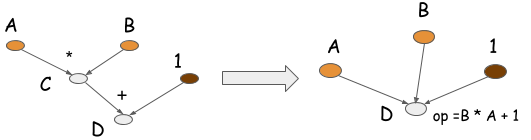• for efficient calculation of the gradient

If for each operator the (partial) derivative is defined, gradients of the computation graph can be computated automatically by backpropagation (application of the chain rule).

#### (Recursive application) of the chain rule¶

Chain rule for a function $c(b(w))$ ($c$ is a function of $b$ and $b$ is a function of $w$):

$$\frac{\partial c(b(w))}{\partial w} = \frac{\partial c(b(w))}{\partial b} \frac{\partial b(w)}{\partial w}$$

#### Sum rule and product rule¶

if $b$ is not a function of $u$:

$$\frac{ \partial (a b)}{\partial u} = \frac{\partial a}{\partial u} b + a \frac{\partial b}{\partial u} = \frac{\partial a}{\partial u} b$$$$\frac{ \partial (a(u) + b)}{\partial u} = \frac{\partial a}{\partial u}$$

### Backpropagation¶

If we have a computational graph to compute $c$ and two parameters in the graph $v$ and $w$, e.g. the following computational graph:

                    o1 (name='c')
/ \
/   \
/     z
/
o2 (name='b')
/ \
/   \
/     y
/
o3 (name='a')
/ \
/   \
w     v

o1,o2 and o3 are arbitrary operators, $y$ and $z$ and branches of the tree.

And we want to compute the two partial derivatives $\frac{\partial c(b(a(w, v)))}{\partial w}$ and $\frac{\partial c(b(a(w, v)))}{\partial v}$.

Note that the application of the chain rule results in:

$$\frac{\partial c(b(a(w, v)))}{\partial w} = \frac{\partial c(b)}{\partial b}\frac{\partial b(a)}{\partial a} \frac{\partial a(w,v)}{\partial w}$$$$\frac{\partial c(b(a(w, v)))}{\partial v} = \frac{\partial c(b)}{\partial b}\frac{\partial b(a)}{\partial a} \frac{\partial a(w,v)}{\partial v}$$

The first two factors of both expressions are the same. So we need to compute them only once. That's the key idea of back propagation. We need to compute such factors only once and can backpropagate them through the graph.

Example for the given operator graph:
Assume e.g. o1 and o3 is a multiplication ($*$) and o2 is an add-Operator ($+$). Application of the chain rule ($z$ is not a function of $w$):

$$\frac{\partial c}{\partial w} = \frac{\partial c(b)}{\partial b}\frac{\partial b(w)}{\partial w} = \frac{\partial (z * b)}{\partial b}\frac{\partial b(w)}{\partial w} = z \frac{\partial (b(w))}{\partial w}$$$$\frac{\partial (b(a(w)))}{\partial w} = \frac{\partial (b(a))}{\partial a} \frac{\partial a(w)}{\partial w}= \frac{\partial (a+y)}{\partial a} \frac{\partial a(w)}{\partial w} = 1 \frac{\partial (a(w))}{\partial w}$$$$\frac{\partial (a(w))}{\partial w} = \frac{\partial (wv)}{\partial w} = v$$

so we have:

$$\frac{\partial c}{\partial w} = z * 1 * v = z * v$$

For $\frac{\partial c}{\partial v}$ the first to factors of the chain rule ('$z$' and '1') are the same and we can reuse it.

$$\frac{\partial c}{\partial v} = z * 1 * \frac{\partial a(v)}{\partial w} = z * 1 * w = z * w$$

#### Example implementation of autodiff (imperative)¶

Reverse mode differentiation.

#### Multivariable Chain Rule¶

$$\frac{\partial}{\partial t} f(x(t), y(t)) = \frac{\partial f}{\partial x} \frac{\partial x}{\partial t}+ \frac{\partial f}{\partial y}\frac{\partial y}{\partial t}$$

If there are multiple paths to a parameter (here $t$), we must add the partial derivatives (sloppy: "the gradients").

Note: We have to add multiple path to the same variable as the multivariable chain rule tells us.

For the implementation we store the partial derivaties in a python dictionary.

We need a helper function combine_dicts for taking the multivariate chain rule into account:

In :
import operator
x = (list(a.items()) + list(b.items()) +
[(k, op(a[k], b[k])) for k in set(b) & set(a)])
return {x[i]: x[i] for i in range(0, len(x))}

In :
a = {'a': 2, 'b':3, 'c':4}
b = {'a': 5, 'c':6, 'x':7}
combine_dicts(a, b)

Out:
{'a': 7, 'b': 3, 'c': 10, 'x': 7}
In :
A = {'a':-1.3, 'b':-4, 'c':3}
B = {'b':3, 'c':4, 'd':5}
combine_dicts(A,B)

Out:
{'a': -1.3, 'b': -1, 'c': 7, 'd': 5}

#### Autodiff class Scalar¶

The deep learning frameworks support automatic differentiation via backpropagation through the computational graph.

To demonstrate how autodiff works we implement a simple autodiff python class Scalar. For each operator we have to implement additionally how the operator is differentiated (grad):

In :
class Scalar(object) :
"""Simple Scalar object that support autodiff."""

def __init__(self, value, name=None):
self.value = value
if name:
self.grad = lambda g : {name : g}
else:
self.grad = lambda g : {}

assert isinstance(other, Scalar)
# forward pass: addition is simple:
ret = Scalar(self.value + other.value)

#
return x

return ret

def __mul__(self, other):
assert isinstance(other, Scalar)
ret = Scalar(self.value * other.value)

#
x = combine_dicts(x, other.grad(g * self.value))
return x
return ret

def square(self):
ret = Scalar(self.value**2)

#
x = self.grad(2.0 * self.value * g)
return x
return ret

                   + (name='d')
/ \
(d./dc = g) /   \
/     1
/
|
* (name='c')
/ \
(d./da = b*g)/   \ (d./db = a*g)
/     \
a=1     b=2

g: backpropagated "gradient value" - in general different at each position
starts at the root of the tree with g = 1  (d root/d root = 1)

Example: $$d = (a * b) + 1$$

$$\frac{\partial d}{\partial a} = \frac{\partial (a*b+1)}{ \partial a} = b$$$$\frac{\partial d}{\partial b} = \frac{\partial (a*b+1)}{ \partial b} = a$$
In :
a = Scalar(1., 'a')
b = Scalar(2., 'b')
c = b * a
d = c + Scalar(1.)
print (d.value)

# backpropagtion of the value 1 through the graph:

3.0
derivatives of d: {'b': 1.0, 'a': 2.0}


#### Example Linear Regression with autodiff class¶

In :
# generate some train data
x_min = -10.
x_max = 10.
m = 10

x = np.random.uniform(x_min, x_max, m)
a = 10.
c = 5.
y_noise_sigma = 3.
y = a + c * x + np.random.randn(len(x)) * y_noise_sigma

In :
%matplotlib inline
import matplotlib.pyplot as plt
plt.plot(x, y, "bo")
plt.xlabel("x")
plt.ylabel("y")

Out:
<matplotlib.text.Text at 0x1152982b0>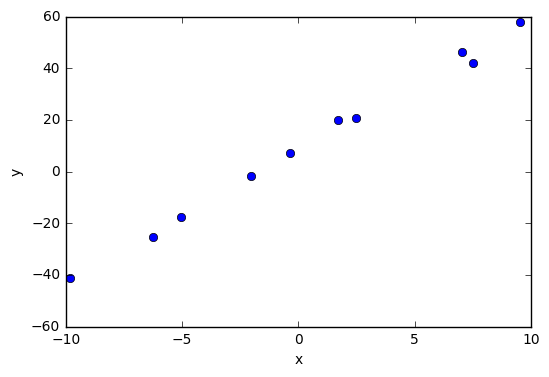In :
# Univariate Linear Regression

# train data
x_values = x
y_values = y

# start values
b_value=15.; w_value=2.

assert x_values.shape == y_values.shape
nb_examples = x_values.shape

def linear_model_with_data(b_value, w_value, x_values, y_values):
b = Scalar(b_value, name='b')
w = Scalar(w_value, name='w')
cost = Scalar(0.)
h = []

for x, y in zip(x_values, y_values):
h_ = b + w * Scalar(x)
diff = h_ + Scalar(-1.) * Scalar(y)
cost = cost + diff.square()
h.append(h_)
cost = cost * Scalar(1./nb_examples)
return h, cost

def get_linear_model(x_values, y_values):
return lambda b_value, w_value: linear_model_with_data(
b_value, w_value, x_values, y_values)

linear_model = get_linear_model(x_values, y_values)

h, cost = linear_model(b_value, w_value)
#print ([i.value for i in h] )
print (cost.value)

382.767059316

In :
# Gradient Descent

learning_rate = 0.01
n_iterations = 200

costs = np.ndarray([n_iterations])
for i in range(n_iterations):
b_value = b_value - learning_rate * deltas['b']
w_value = w_value - learning_rate * deltas['w']
h, cost = linear_model(b_value, w_value)
costs[i] = cost.value

#print ([i.value for i in h] )
print (b_value, w_value)
print (cost.value)

8.48138356481 5.08842430955
3.73616715608

In :
plt.plot(range(n_iterations), costs)
plt.xlabel("Iterations")
plt.ylabel("Cost")

Out:
<matplotlib.text.Text at 0x115406668>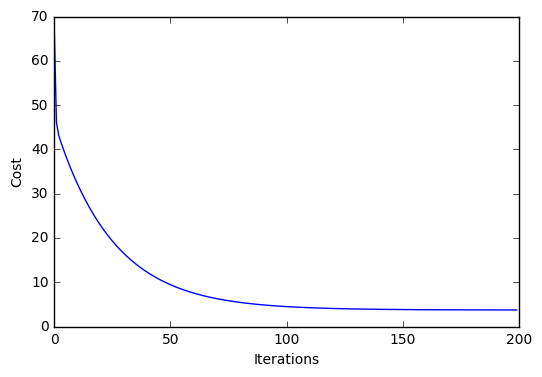In :
plt.plot(x,y, "bo")

xx = np.array((-10.,10))
yy = b_value + w_value * xx
plt.plot(xx, yy, 'r-')
plt.xlabel("x")
plt.ylabel("y")

Out:
<matplotlib.text.Text at 0x115546ac8>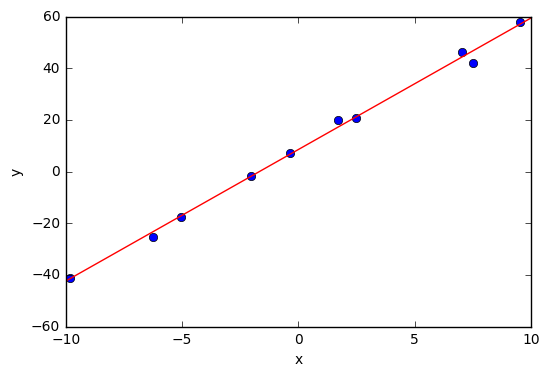Example: Matrix Multiplication

In :
# forward pass
theta = np.random.randn(6, 9)
x = np.random.randn(9, 4)
mprod = np.dot(theta, x) # shape [6, 4]

# gradient backproped down to mprop
grad_mprod = np.random.randn(*mprod.shape) # same shape as mprop

# shape of grad_theta has to be [6, 4]
# shape of grad_x has to be [9,4]


For a more thorough discussion, see [LM] and [Bay15].

### Autodiff for symbolic computation¶

An extra graph for computing the gradient is build.

In :
from IPython.display import Image
Image(filename='pics/comp_graph_backward.png', height=400)

Out: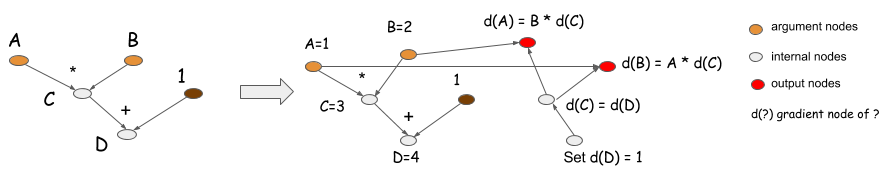In :
# in theano each symbolic variable must be assigned a tensor (shape) type and data type
A = T.dscalar('A') # default of dtype is floatX
print ("A.dtype:", A.dtype)
B = T.dscalar('B')
C = T.mul(B, A)
D = C + 1
f = function(inputs=[A, B], outputs=D) # compile a function

A.dtype: float64

In :
dD_dA, dD_dB = T.grad(D, wrt=[A, B])
from theano import pp
print (pp(dD_dA)) # print out the gradient prior to optimization
print (pp(dD_dB))

(fill(((B * A) + TensorConstant{1}), TensorConstant{1.0}) * B)
(fill(((B * A) + TensorConstant{1}), TensorConstant{1.0}) * A)

In :
grad_a = function(inputs=[A,B], outputs=dD_dA)

In :
grad_a(1,2)

Out:
array(2.0)
In :
grad_b(1,2)

Out:
array(1.0)

In :
# if no graph is specified the default graph is used

A = tf.placeholder(name="A", dtype=tf.float32)
B = tf.placeholder(name="B", dtype=tf.float32)
C = tf.multiply(B, A, name="multiplication")
D = C + 1

In :
help(tf.gradients)

Help on function gradients in module tensorflow.python.ops.gradients_impl:

Constructs symbolic partial derivatives of sum of ys w.r.t. x in xs.

ys and xs are each a Tensor or a list of tensors.  grad_ys
is a list of Tensor, holding the gradients received by the
ys. The list must be the same length as ys.

gradients() adds ops to the graph to output the partial
derivatives of ys with respect to xs.  It returns a list of
Tensor of length len(xs) where each tensor is the sum(dy/dx)
for y in ys.

grad_ys is a list of tensors of the same length as ys that holds
the initial gradients for each y in ys.  When grad_ys is None,
we fill in a tensor of '1's of the shape of y for each y in ys.  A
user can provide their own initial grad_ys to compute the
derivatives using a different initial gradient for each y (e.g., if
one wanted to weight the gradient differently for each value in
each y).

Args:
ys: A Tensor or list of tensors to be differentiated.
xs: A Tensor or list of tensors to be used for differentiation.
grad_ys: Optional. A Tensor or list of tensors the same size as
ys and holding the gradients computed for each y in ys.
name: Optional name to use for grouping all the gradient ops together.
the corresponding op.
for an operations.  This avoids some race conditions.
aggregation_method: Specifies the method used to combine gradient terms.
Accepted values are constants defined in the class AggregationMethod.

Returns:
A list of sum(dy/dx) for each x in xs.

Raises:
LookupError: if one of the operations between x and y does not
ValueError: if the arguments are invalid.


In :
grad = tf.gradients(D, [A, B])
with tf.Session() as sess:
#sess.run(tf.initialize_all_variables())

[3.0, 5.0]


#### Exercise¶

Implement the univariate linear regression with tensorflow. Implement gradient descent with tf.gradients.

Note:

• Parameters of the models are typically implemented with (shared) variables. The gradient calculation with tf.gradients works with variables in the same way as shown above.
• To update a variable use tf.assign:
In :
v = tf.Variable(0., name="v")
c = tf.constant(0.1)
update = tf.assign(v, new_value)
init_op = tf.initialize_all_variables()
with tf.Session() as sess:
sess.run(init_op)
print(sess.run(v))
for _ in range(3):
sess.run(update)
print(sess.run(v))

WARNING:tensorflow:From <ipython-input-43-d32b2d9c04c7>:5: initialize_all_variables (from tensorflow.python.ops.variables) is deprecated and will be removed after 2017-03-02.
Instructions for updating:
Use tf.global_variables_initializer instead.
0.0
0.1
0.2
0.3